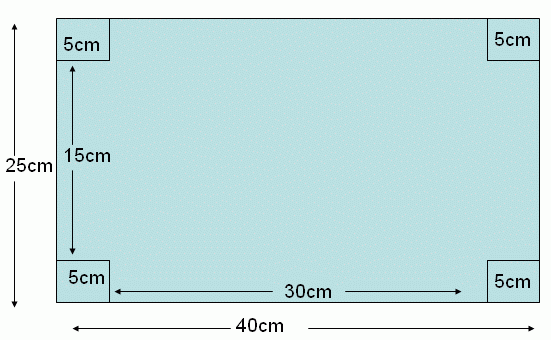Email us to get an instant 20% discount on highly effective K-12 Math & English kwizNET Programs!

Online Quiz (WorksheetABCD)

Questions Per Quiz = 2 4 6 8 10

Grade 7 - Mathematics9.60 Word Problems on Cuboid - I

 Example: The length and width of a rectangular metal sheet are 40 cm and 25 cm respectively. Four squares each of side 5 cm are cut and removed from four corners of the sheet, and the remainder is folded to form a cuboid(without the top face). Find the volume. Solution:In the given rectangular sheet has length and breadth equal to 40 cm and 25 cm respectively. If four corner squares of the side 5 cm are removed and rest is folded into a cuboid. Then the length and width of the cuboid is reduced by twice the side of square. Therefore the length of the cuboid = 40 - (2 * 5) = 40 - 10 length (l)= 30 cm. The width of the cuboid = 25 - (2 * 5) = 25 - 10 width (b) = 15 cm. The height of the cuboid itself is equal to the side of the square removed. Therefore the height of the cuboid = 5 cm. Hence the volume of the cuboid is V = l * b * h V = 30 * 15 * 5 Volume = 2250 cubic centimeters. Directions: Read the above example carefully and answer the following questions. Also make 10 different examples of your own and illustrate each by making cuboids.
 Q 1: The length of a rectangular metal sheet is 35 cm. Four squares each of side 5 cm are cut and removed from four corners of the sheet, and the remainder is folded to form a cuboid(without the top face). Find the width of the rectangular sheet if its volume is given by 2500 cubic centimeters.26 centimeters35 centimeters30 centimeters24 centimeters Q 2: The length and breadth of a rectangular metal sheet are 50 cm and 40 cm respectively. Four squares each of side 'x' cm are cut and removed from four corners of the sheet, and the remainder is folded to form a cuboid(without the top face). Find 'x' if the volume is given by 6384 cubic centimeters.8 centimeters5 centimeters7 centimeters6 centimeters Q 3: The length and width of a rectangular metal sheet are 45 cm & 35 cm respectively. Four squares each of side 'a' cm are cut and removed from four corners of the sheet, and the remainder is folded to form a cuboid(without the top face). Find 'a' if the volume is given by 4488 cubic centimeters.4.5 centimeters5.5 centimeters7.5 centimeters6.5 centimeters Q 4: The length of a rectangular metal sheet are 25 cm. Four squares each of side 4.5 cm are cut and removed from four corners of the sheet, and the remainder is folded to form a cuboid(without the top face). Find the width of the rectangular metal sheet if its volume is given by 792 cubic centimeters.30 centimeters32 centimeters25 centimeters20 centimeters Question 5: This question is available to subscribers only! Question 6: This question is available to subscribers only!